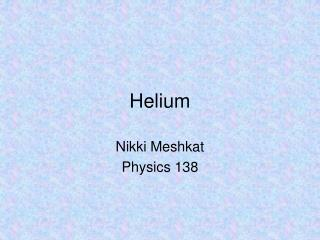DownloadDownload PresentationHelium

# Helium

Télécharger la présentation## Helium

- - - - - - - - - - - - - - - - - - - - - - - - - - - E N D - - - - - - - - - - - - - - - - - - - - - - - - - - -
##### Presentation Transcript

1. Helium Nikki Meshkat Physics 138

2. Outline • Interesting facts about Helium • Solving the Schrodinger Equation for He • Ground State • Excited States • Spin • Solve integrals for various cases

3. Fun Facts about Helium • Helium is named after the Greek god of the sun “Helios” • Discovered on the sun before it was discovered on Earth. Pierre Janssen, a French astronomer, noticed a yellow line in the sun’s spectrum while he was studying a solar eclipse in 1868. Then Sir Norman Lockyer, an English astronomer, realized that this yellow line could not be produced by any known element at the time. He named it Helium. • Helium is produced in stars by fusion of hydrogen. This process creates alpha particles, which is Helium without its electrons.

4. Fun Facts • Helium is the second most abundant element in the universe after Hydrogen • Thermal conductivity is higher than all gases except Hydrogen • Lowest boiling (4.22 K) and melting (0.95 K) points among all elements • Liquid Helium is an important cryogenic material, used to study superconductivity and to cool superconducting magnets. • DOE’s Jefferson Lab uses large amounts of liquid helium to operate its superconductive electron accelerator.

5. Helium created on earth by alpha decay of radioactive elements. The alpha particles trap electrons to become He, which is then trapped in natural gas. There are no known compounds that contain Helium, although attempts are being made to produce Helium Diflouride. When Helium is inhaled, it excites the higher harmonics of the vocal tract, since the speed of sound in He is 3 times that in air. One’s voice is a linear combination of the fundamental frequency plus higher harmonics, so Helium causes there to be more contribution from the higher harmonics to your voice and less contribution from the lower frequencies. More Fun Facts

6. Physics of Helium Let’s look at the Schrodinger Equation with the Hamiltonian for Helium: How can we solve it? Ignore mutual repulsion term!!!

7. First solve an easier problem • Approximate as two Hydrogenic Ions and perform seperation of variables For ground state:

8. What about the repulsion term? Think of it as a perturbation: For example, the ground state has energy:

9. How about the excited states? Let’s look at the case where one electron is in the 1s state and the other electron is in any state (call it nl). But wait, there’s a degeneracy, so we need degenerate perturbation theory! Then the wave function is just a linear combination of these basis functions

10. Plug and chug away!

11. So what does this all mean? Plugging this back in, we get b=a or b= -a

12. Don’t forget spin! Since electrons are fermions, the total wavefunction must be antisymmetric under exchange of particles:

13. Evaluation of Direct Integrals Ground state: Coulomb repulsion between electron clouds The electrostatic potential at r2 produced by electron 1 is: But spherical symmetry means that the charge in acts like a point charge at the origin: where So electrostatic energy from repulsion is The total energy of the repulsion between electrons is twice this since there is an equal contribution to the energy from

14. Is this a good answer? • Recall that total energy without mutual repulsion term was -109 eV • Thus total energy is -109+34=-75eV • To go from singly ionized He to doubly ionized He takes 54.4 eV, thus first ionization energy is 75-54=21eV • Measured ionization energy is 24.6eV • Why wrong answer?

15. Variational Method • Notice that expectation value of perturbation 34eV is not small compared to binding energy 75eV, thus we aren’t completely justified in using perturbation theory. • Better approximation to wave function can be found by using variational method.

16. Evaluation of Direct Integrals Excited state: 1snl Assume the excited electron lies outside the 1s wave function. Then the inner electron screens the outer electron from the full nuclear charge, so that the outer electron feels only 1 proton: Expanding 1/r_12 in terms of spherical harmonics…

17. Evaluation of Exchange Integral • Similar trick with expansion of 1/r_12 term Close to measured value of 0.25eV

18. Conclusion • Helium can be solved by first looking at Hydrogenic case and then adding perturbation term • This method does not give perfect results for the ground state, so the variational method works best in this case • Methods used for Helium can be extended to solving many-electron atoms